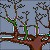## Purpose of LDA

The purpose of Discriminant Analysis is to classify objects (people, customers, things, etc.) into one of two or more groups based on a set of features that describe the objects (e.g. gender, age, income, weight, preference score, etc. ). In general, we assign an object to one of a number of predetermined groups based on observations made on the object.

Note that the groups are known or predetermined and do not have order (i.e. nominal scale). The classification problem gives several objects with a set features measured from those objects. What we are looking for is two things:

1. Which set of features can best determine group membership of the object?
2. What is the classification rule or model to best separate those groups?

The first purpose is feature selection and the second purpose is classification. In this tutorial we will not cover the first purpose (reader interested in this step wise approach can use statistical software such as SPSS, SAS or statistical package of Matlab. However, we do cover the second purpose to get the rule of classification and predict new object based on the rule.

## Linear Discriminant Analysis

For example, we want to know whether a soap product is good or bad based on several measurements on the product such as weight, volume, people's preferential score, smell, color contrast etc. The object here is soap. The class category or the group ("good" and "bad") is what we are looking for (it is also called dependent variable). Each measurement on the product is called features that describe the object (it is also called independent variable).

Thus, in discriminant analysis, the dependent variable (Y) is the group and the independent variables (X) are the object features that might describe the group. The dependent variable is always category (nominal scale) variable while the independent variables can be any measurement scale (i.e. nominal, ordinal, interval or ratio).

If we can assume that the groups are linearly separable, we can use linear discriminant model (LDA). Linearly separable suggests that the groups can be separated by a linear combination of features that describe the objects. If only two features, the separators between objects group will become lines. If the features are three, the separator is a plane and the number of features (i.e. independent variables) is more than 3, the separators become a hyper-plane.

## LDA Formula

Using classification criterion to minimize total error of classification (TEC), we tend to make the proportion of object that it misclassifies as small as possible. TEC is the performance rule in the 'long run' on a random sample of objects. Thus, TEC should be thought as the probability that the rule under consideration will misclassify an object. The classification rule is to a ssign an object to the group with highest conditional probability . This is called Bayes Rule. This rule also minimizes the TEC. If there are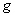groups, the Bayes' rule is to assign the object to group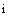where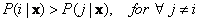.

We want to know the probability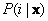that an object is belong to group, given a set of measurement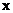. In practice however, the quantity ofis difficult to obtain. What we can get is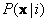. This is the probability of getting a particular set of measurementgiven that the object comes from group. For example, after we know that the soap is good or bad then we can measure the object (weight, smell, color etc.). What we want to know is to determine the group of the soap (good or bad) based on the measurement only.

Fortunately, there is a relationship between the two conditional probabilities that well known as Bayes Theorem: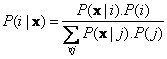Prior probability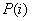is probability about the group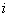known without making any measurement. In practice we can assume the prior probability is equal for all groups or based on the number of sample in each group.

In practice, however, to use the Bayes rule directly is unpractical because to obtainneed so much data to get the relative frequencies of each groups for each measurement. It is more practical to assume the distribution and get the probability theoretically. If we assume that each group has multivariate Normal distribution and all groups have the same covariance matrix, we get what is called Linear Discriminant Analysis formula: (see: Derivation of this formula here )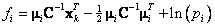Assign object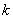to groupthat has maximum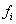If you notice carefully the second term (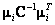) is actually Mahalanobis distance , which is distance to measure dissimilarity between several groups.

Any standard text books in data mining, pattern recognition or classification can give you more detail derivation of this formula. The meaning of each variable is explained in the next section of numerical example .

Preferable reference for this tutorial is

Teknomo, Kardi (2015) Discriminant Analysis Tutorial. http://people.revoledu.com/kardi/ tutorial/LDA/